Here we visualize filters and outputs using the network architecture proposed by Krizhevsky et al. for ImageNet and implemented in caffe.

First, import required modules and set plotting parameters

In :
import numpy as np
import matplotlib.pyplot as plt
%matplotlib inline

# Make sure that caffe is on the python path:
caffe_root = '../'  # this file is expected to be in {caffe_root}/examples
import sys
sys.path.insert(0, caffe_root + 'python')

import caffe

plt.rcParams['figure.figsize'] = (10, 10)
plt.rcParams['image.interpolation'] = 'nearest'
plt.rcParams['image.cmap'] = 'gray'


Follow the instructions for getting the pretrained models, load the net, specify test phase and CPU mode, and configure input preprocessing.

In :
net = caffe.Classifier(caffe_root + 'examples/imagenet/imagenet_deploy.prototxt',
caffe_root + 'examples/imagenet/caffe_reference_imagenet_model')
net.set_phase_test()
net.set_mode_cpu()
# input preprocessing: 'data' is the name of the input blob == net.inputs
net.set_mean('data', caffe_root + 'python/caffe/imagenet/ilsvrc_2012_mean.npy')  # ImageNet mean
net.set_channel_swap('data', (2,1,0))  # the reference model has channels in BGR order instead of RGB
net.set_input_scale('data', 255)  # the reference model operates on images in [0,255] range instead of [0,1]


Run a classification pass

In :
scores = net.predict([caffe.io.load_image(caffe_root + 'examples/images/cat.jpg')])


The layer features and their shapes (10 is the batch size, corresponding to the the ten subcrops used by Krizhevsky et al.)

In :
[(k, v.data.shape) for k, v in net.blobs.items()]

Out:
[('data', (10, 3, 227, 227)),
('conv1', (10, 96, 55, 55)),
('pool1', (10, 96, 27, 27)),
('norm1', (10, 96, 27, 27)),
('conv2', (10, 256, 27, 27)),
('pool2', (10, 256, 13, 13)),
('norm2', (10, 256, 13, 13)),
('conv3', (10, 384, 13, 13)),
('conv4', (10, 384, 13, 13)),
('conv5', (10, 256, 13, 13)),
('pool5', (10, 256, 6, 6)),
('fc6', (10, 4096, 1, 1)),
('fc7', (10, 4096, 1, 1)),
('fc8', (10, 1000, 1, 1)),
('prob', (10, 1000, 1, 1))]

The parameters and their shapes (each of these layers also has biases which are omitted here)

In :
[(k, v.data.shape) for k, v in net.params.items()]

Out:
[('conv1', (96, 3, 11, 11)),
('conv2', (256, 48, 5, 5)),
('conv3', (384, 256, 3, 3)),
('conv4', (384, 192, 3, 3)),
('conv5', (256, 192, 3, 3)),
('fc6', (1, 1, 4096, 9216)),
('fc7', (1, 1, 4096, 4096)),
('fc8', (1, 1, 1000, 4096))]

Helper functions for visualization

In :
# our network takes BGR images, so we need to switch color channels
def showimage(im):
if im.ndim == 3:
im = im[:, :, ::-1]
plt.imshow(im)

# take an array of shape (n, height, width) or (n, height, width, channels)
#  and visualize each (height, width) thing in a grid of size approx. sqrt(n) by sqrt(n)
data -= data.min()
data /= data.max()

# force the number of filters to be square
n = int(np.ceil(np.sqrt(data.shape)))
padding = ((0, n ** 2 - data.shape), (0, padsize), (0, padsize)) + ((0, 0),) * (data.ndim - 3)

# tile the filters into an image
data = data.reshape((n, n) + data.shape[1:]).transpose((0, 2, 1, 3) + tuple(range(4, data.ndim + 1)))
data = data.reshape((n * data.shape, n * data.shape) + data.shape[4:])

showimage(data)


The input image

In :
# index four is the center crop
image = net.blobs['data'].data.copy()
image -= image.min()
image /= image.max()
showimage(image.transpose(1, 2, 0))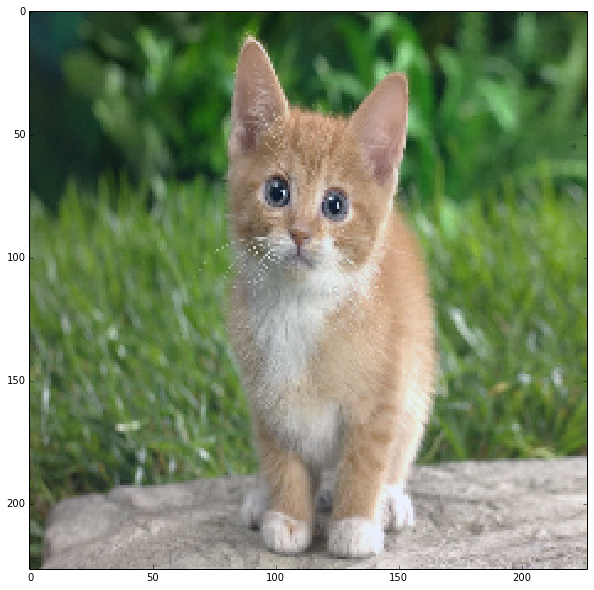The first layer filters, conv1

In :
# the parameters are a list of [weights, biases]
filters = net.params['conv1'].data
vis_square(filters.transpose(0, 2, 3, 1))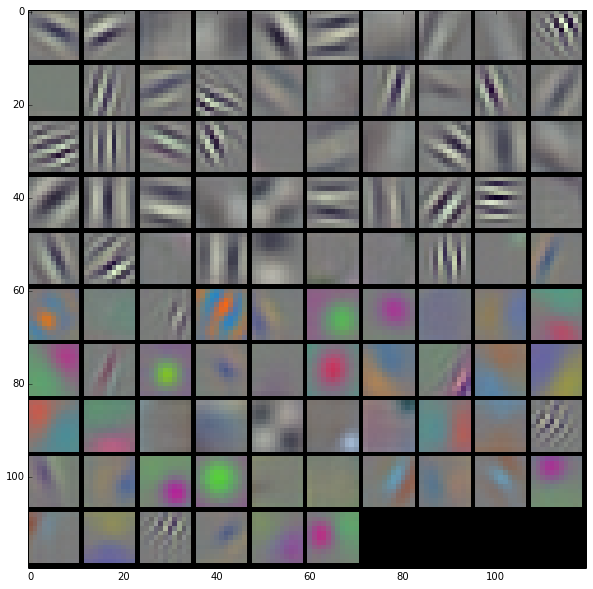The first layer output, conv1 (rectified responses of the filters above, first 36 only)

In :
feat = net.blobs['conv1'].data[4, :36]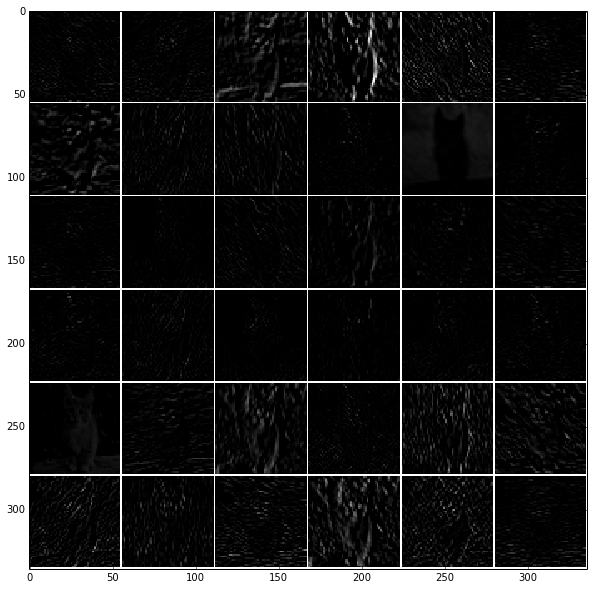The second layer filters, conv2

There are 128 filters, each of which has dimension 5 x 5 x 48. We show only the first 48 filters, with each channel shown separately, so that each filter is a row.

In :
filters = net.params['conv2'].data
vis_square(filters[:48].reshape(48**2, 5, 5))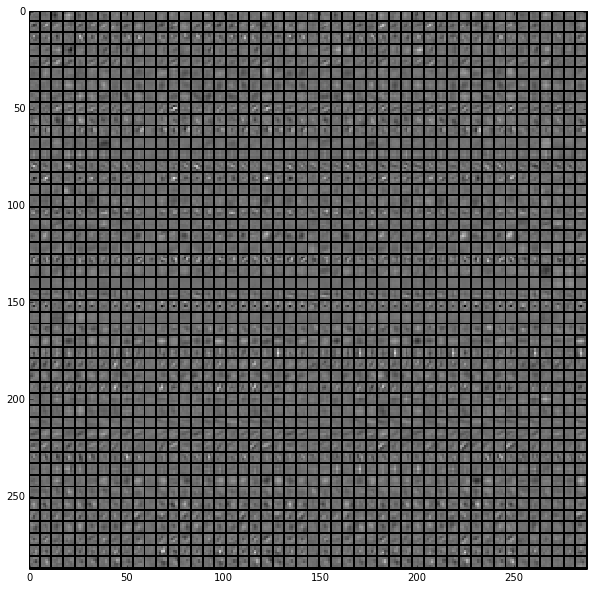The second layer output, conv2 (rectified, only the first 36 of 256 channels)

In :
feat = net.blobs['conv2'].data[4, :36]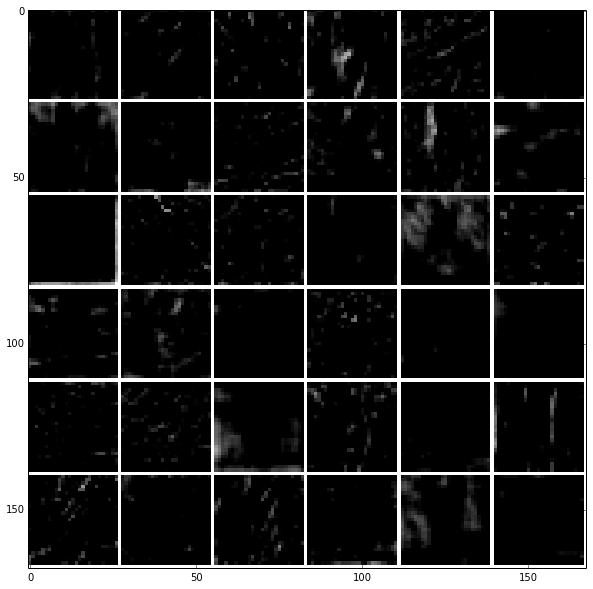The third layer output, conv3 (rectified, all 384 channels)

In :
feat = net.blobs['conv3'].data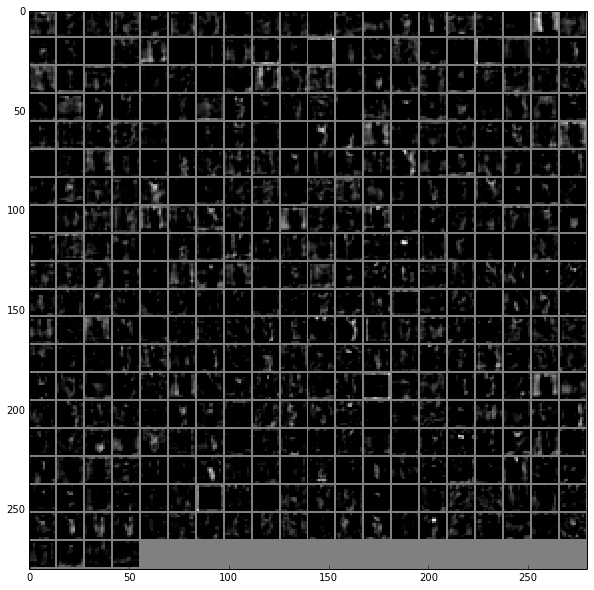The fourth layer output, conv4 (rectified, all 384 channels)

In :
feat = net.blobs['conv4'].data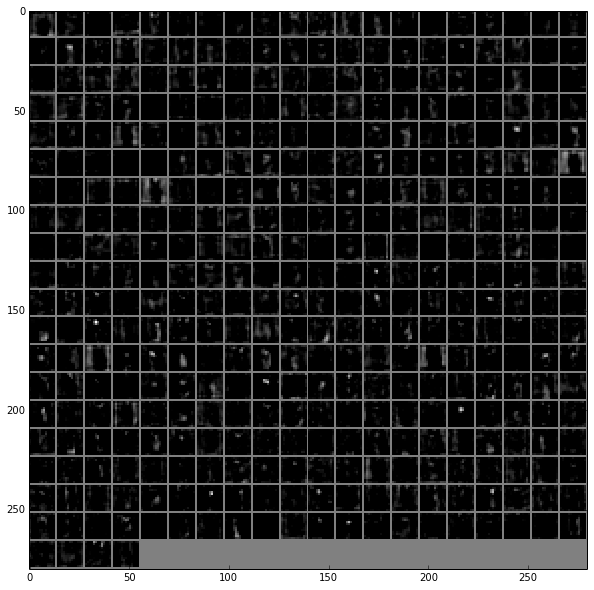The fifth layer output, conv5 (rectified, all 256 channels)

In :
feat = net.blobs['conv5'].data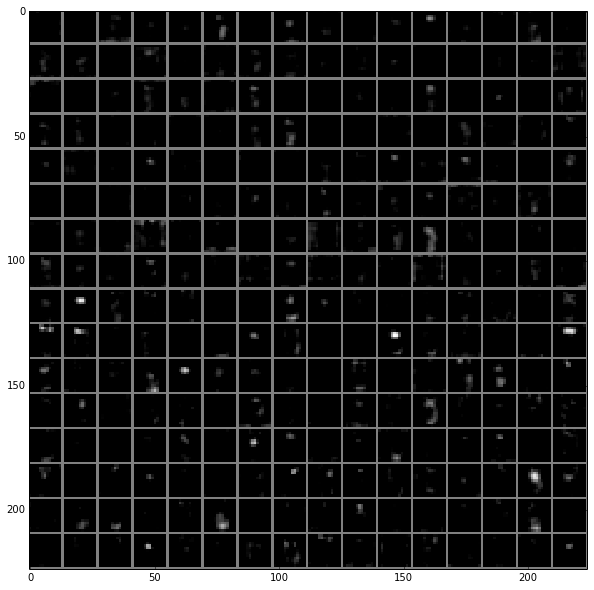The fifth layer after pooling, pool5

In :
feat = net.blobs['pool5'].data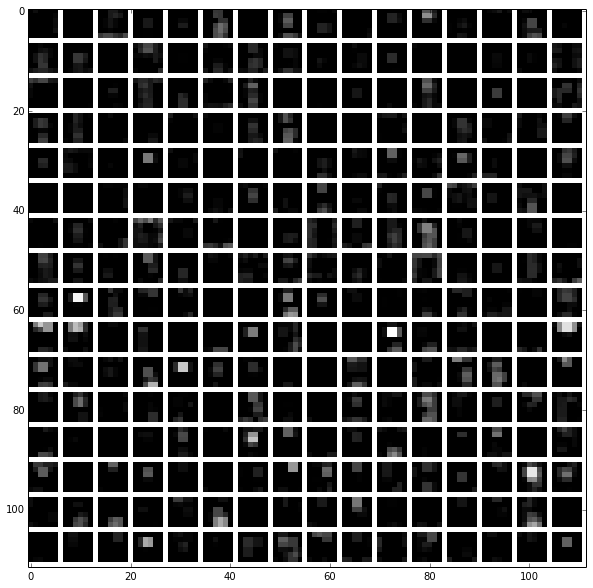The first fully connected layer, fc6 (rectified)

We show the output values and the histogram of the positive values

In :
feat = net.blobs['fc6'].data
plt.subplot(2, 1, 1)
plt.plot(feat.flat)
plt.subplot(2, 1, 2)
_ = plt.hist(feat.flat[feat.flat > 0], bins=100)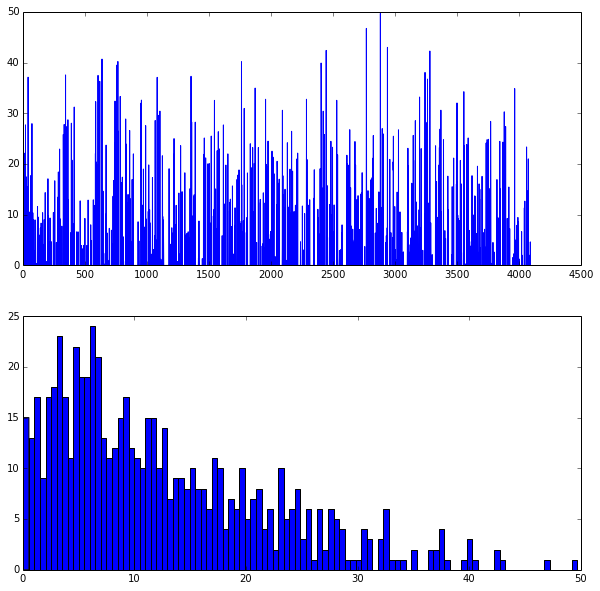The second fully connected layer, fc7 (rectified)

In :
feat = net.blobs['fc7'].data
plt.subplot(2, 1, 1)
plt.plot(feat.flat)
plt.subplot(2, 1, 2)
_ = plt.hist(feat.flat[feat.flat > 0], bins=100)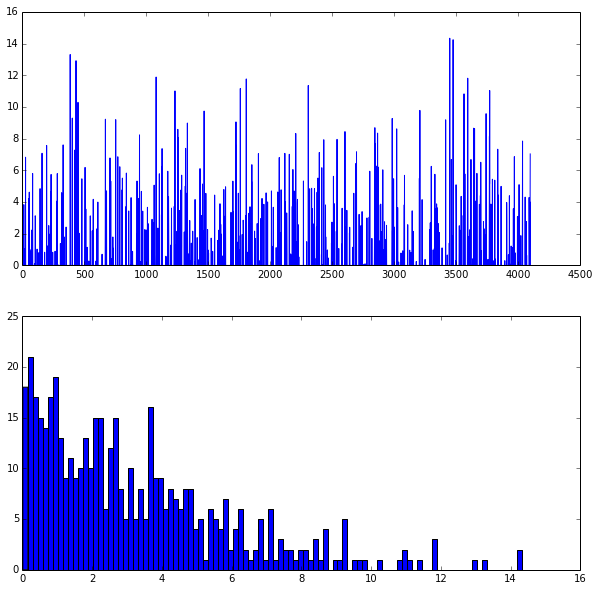The final probability output, prob

In :
feat = net.blobs['prob'].data
plt.plot(feat.flat)

Out:
[<matplotlib.lines.Line2D at 0x12b260710>]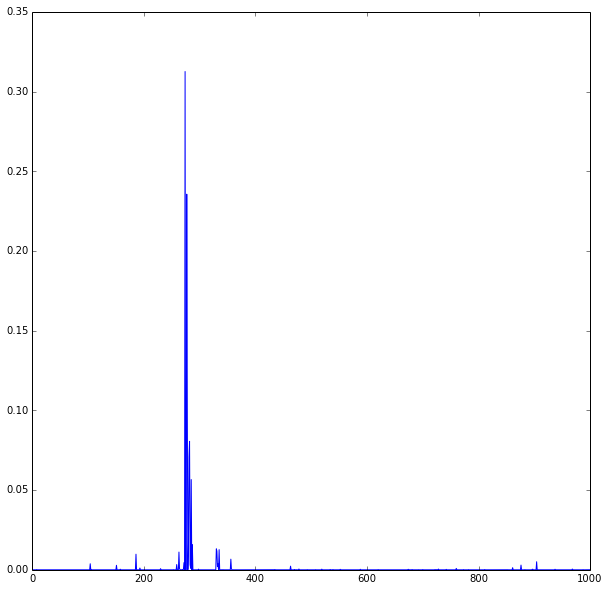Let's see the top 5 predicted labels.

In :
imagenet_labels_filename = caffe_root + 'data/ilsvrc12/synset_words.txt'
try:

top_k = net.blobs['prob'].data.flatten().argsort()[-1:-6:-1]

['n02115913 dhole, Cuon alpinus' 'n02119022 red fox, Vulpes vulpes'